## Aspire's Library

A Place for Latest Exam wise Questions, Videos, Previous Year Papers,
Study Stuff for MCA Examinations

## NIMCET Previous Year Questions (PYQs)

#### NIMCET Trigonometry PYQ

NIMCET PYQ
The expression  $\frac{tanA}{1-cotA}+\frac{cotA}{1-tanA}$ can be written as

NIMCET Previous Year PYQNIMCET NIMCET 2020 PYQ

#### SolutionNIMCET PYQ
Angle of elevation of the top of the tower from 3 points (collinear) A, B and C on a road leading to the foot of the tower are 30°, 45° and 60°, respectively. The ratio of AB and BC is

NIMCET Previous Year PYQNIMCET NIMCET 2020 PYQ

#### Solution

According to the given information, the figure should be as follows.
Let the height of tower = h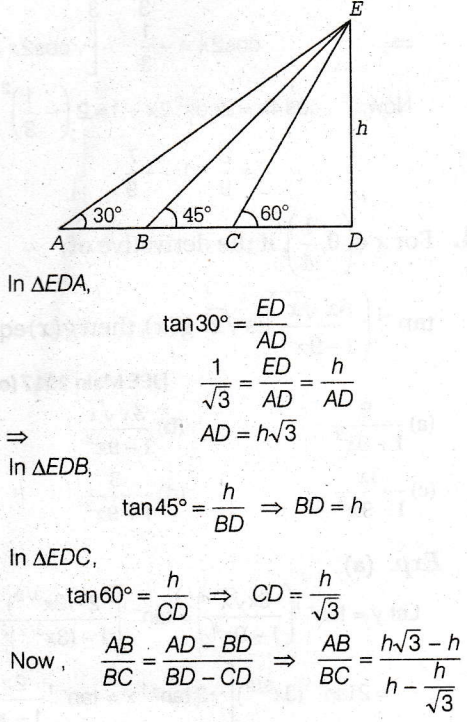NIMCET PYQ
If $3 sin x + 4 cos x = 5$, then $6tan\frac{x}{2}-9tan^2\frac{x}{2}$

NIMCET Previous Year PYQNIMCET NIMCET 2020 PYQ

#### Solution

NIMCET PYQ
Largest value of $cos^2\theta -6sin\theta cos\theta+3sin^2\theta+2$ is

NIMCET Previous Year PYQNIMCET NIMCET 2023 PYQ

#### Solution

NIMCET PYQ
Number of point of which f(x) is not differentiable $f(x)=|cosx|+3$ in $[-\pi, \pi]$

NIMCET Previous Year PYQNIMCET NIMCET 2023 PYQ

#### Solution

NIMCET PYQ
If A > 0, B > 0 and A + B = $\frac{\pi}{6}$ , then the minimum value of $tanA + tanB$

NIMCET Previous Year PYQNIMCET NIMCET 2019 PYQ

#### Solution

On differentiating
x= tanA + tan(π/6-A)
we get :
dx/dA = sec²A-sec²(π/6-A)
now putting
dx/dA=0
we get
cos²(A) = cos²(π/6-A) so 0≤A≤π/6
therefore
A=π/6-A from here we get A = π/12 = B
so minimum value of that function is
2tanπ/12 which is equal to 2(2-√3)

NIMCET PYQ
The $sin^2 x tanx + cos^2 x cot x-sin2x=1+tanx+cotx$, $x \in (0 , \pi)$, then x

NIMCET Previous Year PYQNIMCET NIMCET 2019 PYQ

#### Solution

NIMCET PYQ
If $cosec\theta-\cot \theta=2$, then the value of $cosec\theta$ is

NIMCET Previous Year PYQNIMCET NIMCET 2022 PYQ

#### Solution

NIMCET PYQ
The solution of the equation ${4\cos }^2x+6{\sin }^2x=5$ are

NIMCET Previous Year PYQNIMCET NIMCET 2022 PYQ

#### Solution

NIMCET PYQ
If $a_1, a_2, a_3,...a_n$, are in Arithmetic Progression with common difference d, then the sum $(sind) (cosec a_1 . cosec a_2+cosec a_2.cosec a_2+...+cosec a_{n-1}.cosec a_n)$ is equal to

NIMCET Previous Year PYQNIMCET NIMCET 2022 PYQ

#### Solution

NIMCET PYQ
In a ΔABC, if $\tan ^2\frac{A}{2}+\tan ^2\frac{B}{2}+\tan ^2\frac{C}{2}=k$ , then k is always

NIMCET Previous Year PYQNIMCET NIMCET 2021 PYQ

#### Solution

NIMCET PYQ
The general value of $\theta$, satisfying the equation $\sin \theta=\frac{-1}{2},\, \tan \theta=\frac{1}{\sqrt[]{3}}$

NIMCET Previous Year PYQNIMCET NIMCET 2021 PYQ

#### Solution

NIMCET PYQ
If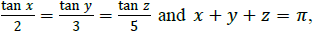then the value of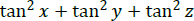is

NIMCET Previous Year PYQNIMCET NIMCET 2018 PYQ

#### Solution

NIMCET PYQ
If tan x = - 3/4 and 3π/2 < x < 2π, then the value of sin2x is

NIMCET Previous Year PYQNIMCET NIMCET 2017 PYQ

#### Solution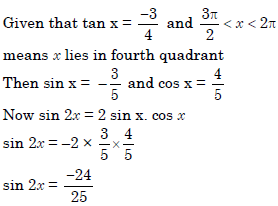NIMCET PYQ
The value of $\tan 9{^{\circ}}-\tan 27{^{\circ}}-\tan 63{^{\circ}}+\tan 81{^{\circ}}$ is equal to

NIMCET Previous Year PYQNIMCET NIMCET 2021 PYQ

#### Solution

NIMCET PYQ
If cosθ = 4/5 and cosϕ = 12/13, θ and ϕ both in the fourth quadrant, the value of cos( θ + ϕ )is

NIMCET Previous Year PYQNIMCET NIMCET 2017 PYQ

#### Solution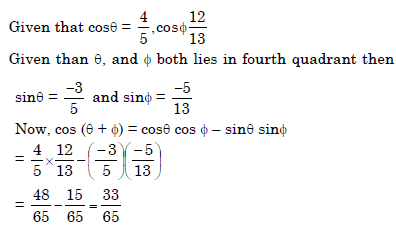NIMCET PYQ
The value of sin36o is

NIMCET Previous Year PYQNIMCET NIMCET 2017 PYQ

#### Solution

NIMCET PYQ
Express (cos 5x – cos7x) as a product of sines or cosines or sines and cosines,

NIMCET Previous Year PYQNIMCET NIMCET 2017 PYQ

#### Solution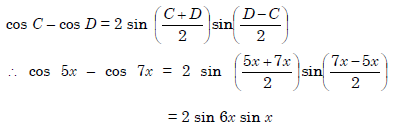NIMCET PYQ
If $32\, \tan ^8\theta=2\cos ^2\alpha-3\cos \alpha$ and $3\, \cos \, 2\theta=1$, then the general value of $\alpha$ =

NIMCET Previous Year PYQNIMCET NIMCET 2021 PYQ

#### Solution

NIMCET PYQ
If |k|=5 and 0° ≤ θ ≤ 360°, then the number of distinct solutions of 3cos⁡θ + 4sin⁡θ = k is
NIMCET 2021

NIMCET Previous Year PYQNIMCET NIMCET 2021 PYQ

#### Solution

NIMCET PYQ
If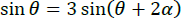, then value of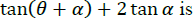NIMCET Previous Year PYQNIMCET NIMCET 2018 PYQ

#### Solution

NIMCET PYQ
If $a\, \cos \theta+b\, \sin \, \theta=2$ and $a\, \sin \, \theta-b\, \cos \, \theta=3$ , then ${a}^{2^{}}+{b}^2=$

NIMCET Previous Year PYQNIMCET NIMCET 2021 PYQ

#### Solution

NIMCET PYQ
If $\prod ^n_{i=1}\tan ({{\alpha}}_i)=1\, \forall{{\alpha}}_i\, \in\Bigg{[}0,\, \frac{\pi}{2}\Bigg{]}$ where i=1,2,3,...,n. Then maximum value of $\prod ^n_{i=1}\sin ({{\alpha}}_i)$.

NIMCET Previous Year PYQNIMCET NIMCET 2023 PYQ

#### Solution

NIMCET PYQ
Solve the equation sin2 x - sinx - 2 = 0 for for x on the interval 0 ≤ x < 2π

NIMCET Previous Year PYQNIMCET NIMCET 2020 PYQ

#### Solution

NIMCET PYQ
If $\frac{tanx}{2}=\frac{tanx}{3}=\frac{tanx}{5}$ and x + y + z = π, then the value of tan2x + tan2y + tan2z is

NIMCET Previous Year PYQNIMCET NIMCET 2020 PYQ

#### Solution

NIMCET PYQ
Find the value of sin 12°sin 48°sin 54°

NIMCET Previous Year PYQNIMCET NIMCET 2020 PYQ

#### Solution

NIMCET PYQ
If cos x = tan y , cot y = tan z and cot z = tan x, then sinx =

NIMCET Previous Year PYQNIMCET NIMCET 2020 PYQ

#### Solution

NIMCET PYQ
The value of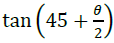is

NIMCET Previous Year PYQNIMCET NIMCET 2020 PYQ

#### SolutionNIMCET PYQ
The value of sin 10°sin 50°sin 70° is

NIMCET Previous Year PYQNIMCET NIMCET 2020 PYQ

#### Solution

sin10° sin50° sin70°
= sin10° sin(60°−10°) sin(60°+10°)
= 1/4 sin3x10°
=1/4x1/2=1/8

#### NIMCET

Online Test Series,
and More.

#### NIMCET

Online Test Series,